﻿ A New Approach in Design of Model-free Fuzzy Sliding Mode Controller for Multivariable Chemical Processes

### A New Approach in Design of Model-free Fuzzy Sliding Mode Controller for Multivariable Chemical Proc...OPEN ACCESSPEER-REVIEWED

## A New Approach in Design of Model-free Fuzzy Sliding Mode Controller for Multivariable Chemical Processes

Mohammad Khodadadi1,, Mahdi Shahbazian1, Masoud Aghajani2

1Department of Instrumentation and Automation Engineering, Ahwaz Faculty of Petroleum, PUT, Ahwaz, Iran

2Department of Chemical Engineering, Ahwaz Faculty of Petroleum, PUT, Ahwaz, Iran

### Abstract

Sliding Mode Control (SMC) as a mode of the Variable Structure Control (VSC) is known as an excellent robust control strategy in the field of uncertain and nonlinear control with high performance. However, chattering and process model dependency as main problems of the original SMC constrain its application. Also, in multivariable nonlinear process control, the interaction among the manipulated and controlled variables can degrade the performance of the controller. The objective of this paper is to design a new model-free Fuzzy Sliding Mode Control (FSMC) to solve these problems in MIMO process control. In this strategy, the equivalent –like term of the SMC’s control law is estimated using a self-tuned MIMO fuzzy logic inference system in order to overcome the dependency of the SMC to the process model and to reject undesirable effect of interaction toward improvement of robustness. Moreover, in design of linear sliding variables for switching term of the SMC, all states error are associated to lessen the interaction effect. Finally, the proposed strategy has been evaluated through implementation on a warm water tank system equipped with two manipulated servo valve to control temperature and water level. This system is nonlinear and affected by unknown disturbances. The results reveal that the purposed scheme is superior in servo tracking and unknown disturbance rejection performances in comparison with the classical PI control.

### At a glance: Figures

123
Prev Next

• Khodadadi, Mohammad, Mahdi Shahbazian, and Masoud Aghajani. "A New Approach in Design of Model-free Fuzzy Sliding Mode Controller for Multivariable Chemical Processes." Journal of Automation and Control 3.1 (2015): 29-35.
• Khodadadi, M. , Shahbazian, M. , & Aghajani, M. (2015). A New Approach in Design of Model-free Fuzzy Sliding Mode Controller for Multivariable Chemical Processes. Journal of Automation and Control, 3(1), 29-35.
• Khodadadi, Mohammad, Mahdi Shahbazian, and Masoud Aghajani. "A New Approach in Design of Model-free Fuzzy Sliding Mode Controller for Multivariable Chemical Processes." Journal of Automation and Control 3, no. 1 (2015): 29-35.

 Import into BibTeX Import into EndNote Import into RefMan Import into RefWorks

### 1. Introduction

Sliding Mode Control (SMC) is a high performance robust control strategy with simple procedure for control of systems whose dynamics are highly nonlinear and uncertain [1, 2, 3]. Hence, this methodology has been developed during last three decades in the nonlinear control area. The SMC control law has two components; continuous and switching (discontinuous) elements . The switching element has different gain signs across the predefined sliding surface. This surface divides the phase plane into two regions where the SMC’s control action has different structure such that its structure is allowed to change alternatively in order to drive the system’s state trajectory onto the manifold-like sliding surface toward the plane’s origin. [3, 4]. During there stricted sliding motion of the system’s state trajectory, its motion will robust against modeling uncertainties and disturbances . However, in original SMC, the problems of chattering and model dependency can restrict its applications . “Chattering phenomenon” is the high frequency oscillation of the state trajectory around the sliding surface because of non-ideal (delayed) switching or non-instantaneous actuating [2, 6] which also can cause extreme heat loss and wearing of the moving parts in instrumentation devices . the boundary layer (BL) approach is as common smoothing technique to filter the control signal to lessen or eliminate the chattering but at cost of robustness specially, in the presence of the large range of uncertainties.  in which the B.L. results in considerable steady state error [8, 9]. To derive he continuous element of the SMC, the model of the plant (relation between control and manipulated variables)is required but it is difficult to use the high order complex model or it is hard to modeling in the complex plants or in the lack of some process parametric knowledge. [5, 10, 11, 12]. Fuzzy Logic Control strategy (FLC) based on fuzzy set theory  is used to design the control law in a form of inferential rules using some qualitative knowledge about the process under control. This model free method is implemented generally to solve the model dependency problem and improvement of control system’s performance . Sliding Mode Fuzzy Control (SMFC) and Fuzzy Sliding Mode Control (FSMC)  are as two integration strategy with features of both original SMC and FLC to remedy the problems of the original SMC which have been widely investigated in recent years such as . The aim of this paper is to design a novel model free-FSMC which can be specially implemented inmulti-variables process control to improve the robustness. For this purpose, a fuzzy continuous element with auto adjustable parameters is proposed to remedy the model dependency problem and removing of steady state error. Furthermore, the sliding variables are developed for each control action using error of all states by consideration the effect of each control action on each state errors to avoid the interaction (likes dynamics decoupling). This paper is organized as follows: section 2 presents the SMC briefly; section 3 illustrates the new model-free FSMC methodology for MIMO processes; in section 4, the proposed FSMC is implemented and its capability is verified; eventually, section 5 concludes the paper.

### 2. A Brief Review of original SMC

Let an nth order single input nonlinear system defined as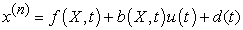(1)

Where,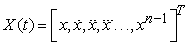is the state vector and scalar x is the control variable. u(t) is the control action.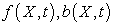are generally uncertain nonlinear functions are estimated as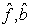and bounded by continuous functions as follows: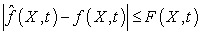(2)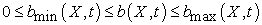(3)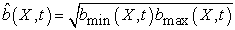(4)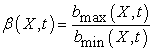(5)

Also, the disturbance d(t) is upper bounded as follows: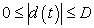(6)

sliding variable [7, 16] is predefined in the state space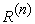as: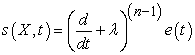(7)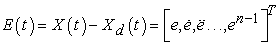(8)

Here,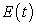is the tracking error vector.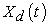is the desired state vector and λ is strictly positive tuning parameter. In second order systems (n=2), Eq.(7) yields the sliding variable as: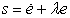(9)

In order to move the error vector on sliding surface (null value sliding variable) and to retain on it, the SMC’s control law is given as follows which is derived based on reaching condition :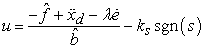(10)

Here, first component is the continuous (equivalent) control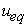and second is discontinuous (switching) control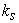respectively .is the positive switching gain  bounded as follows: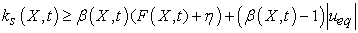(11)

Because of existence of non-ideal switching(restricted frequency and non-instantaneous control switching) in practice due to discretized implementation or neglected high frequency un-modeled dynamics (time delays, actuator’s dynamics,…) [1, 2, 6, 7, 18], the classical SMC controller exhibit oscillatory motion state trajectory with high frequency about the sliding surface so called chattering as depicted in Figure 1. The Boundary Layer technique  is one of unti-chatter methods by mechanism of alleviation of the control signal in the thin layer with thickness of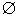around the sliding surface as follows but at cost of tracking error [2, 19]: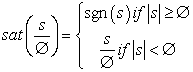(11)
Figure 1. Chattering phenomenon as a result of non-ideal switching 

In the systems with large range of uncertainties and disturbances, the switching gain must be chosen high enough but to cancel the chattering induced by this high gain and the thicknessmust be chosen large enough which can cause considerable off-set .

### 3. The Proposed FSMC

In this section, we attempt to design a novel model-free FSMC strategy for MIMO systems of control. Firstly, the MISO equivalent-like control of each MISO SMC controllers are designed. They are estimated by auto adjustable MISO fuzzy inferential systems to reject uncertainties, whereby the tracking error which appeared due to boundary layer technique will be eliminated. Secondly, new approach is introduced to design sliding variable using error of all states for each sliding variables for canceling of interaction’s effect. The proposed FSMC controllers are constructed as follows (see Figure 2):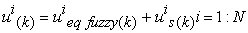(12)

Where,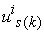,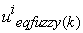and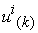are the switching control, fuzzy equivalent-like control and the FSMC output signal as the process input respectively in relevant to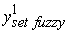manipulated control and N is the number of manipulated variables.

Figure 2. Model free -FSMC controller’s elements: Fuzzy Logic Dynamics Estimator (FLDE) and Switching Control (SC) for each manipulated variable
3.1. Equivalent Control Design

Formulation of the equivalent part of SMC controller will be hard or impossible if the model of the chemical process is complex or ill-defined (with unknown states) or if some recognized states are too expensive to be measured (such as concentration). To solve this model dependency problem, the chemical process can be modeled approximately such as First Order Plus Dead Time (FOPDT)  and it is expected the SMC to be robust in the face of the un-modeled uncertainties which is in this approximated dynamics . It is note that, in the presence of the large range of uncertainties and disturbances, the performance of SMC will be degraded. In this sub-section it is attempted to estimate the equivalent part of the each SMCs in MIMO system by fuzzy logic inference system to solve the model dependency not at cost of robustness.

3.1.1. The Proposed Methodology

To estimate the equivalent control ueq for each manipulated variable, a Multi inputs-Single outputs Fuzzy Logic Dynamic Estimator (FLDE) is established using knowledge about system’s behavior on the steady state condition. The steady-state I/O data of process under control are assigned to centers of O/I membership functions as depicted in Figure 3. This method is development of the method which has been verified for SISO process control in previous work .

3.1.2. FLDE Design Procedure

To design the FLDE, the steady-state operating points of process (outputs/inputs data) are used which are obtained from expert knowledge. ForanN input N output process, each FLDE appropriated to a manipulated variable has N dimensional input and one dimensional output. To design its input membership functions, each dimension appropriated to one of the control variables. Centers of membership functions in each dimension (defined with 4 labels of zero, small, medium and big) are four steady state values of corresponded control variable. Also, the steady state values of each manipulated variables are used as centers of output membership functions of related FLDE. Triangular Membership Functions (MF) are chosen for the fuzzy variables.

In the case of two input-two output process, we have two dimensional rule base for each FLDE are given in Table 1, for instance, two typical rules are as follows: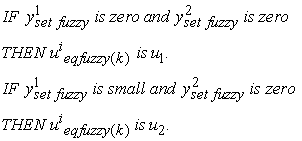(13)

Mamdani product inference engine and Center Average Defuzzifier are used for FLDE estimator. Input and output fuzzy sets are as follows:

Input fuzzy setsfor ith controlled variable: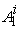=Zero;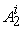= Small;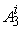=Medium;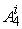=Big; i=1:N

for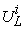controlled variable.

Output fuzzy sets in relevant to ith controlled variable: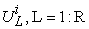Where, R is the number of rules.

Step II) FLDE Self- Tuning

The FLDE estimator is adjusted online based on evanesce of the difference between output of the SMC and output of its equivalent part (FLDE) due to existence of uncertainties such that it is eliminated at steady state condition. The centers of output membership functions of the each FLDEs (as free parameters) can be adjusted online and is done once in each sampling interval as follows (see Figure 3):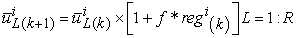(14)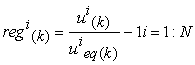(15)

Where,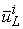is the center in relevant to Lth rule and ith manipulated variable. Also, k is the time-interval at time kTs and Ts is the sampling time. f is fixed step size. In the reaching mode, the center tuners will try to update each FLDEs and reject their uncertainties to achieve zero steady state errors.

#### Table 1. Two dimensional Fuzzy rule base for FLDE1 and FLDE2. Linguistic variables: B=big, M=medium, S=small, Z=zero

3.2. Design of Switching Control

To design the Switching Control (SC) of the proposed FSMC, the new approach is considered in construction of the sliding variable inspired from dynamic decupling method  for cancellation of the interaction effects in control system as follows: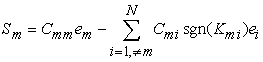(16)

Here,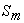is the sliding variable in relevant to the mth manipulated variable whose second term (term) is devised for reduction or elimination of the influence of the other manipulated variables on the mth control variable (interaction).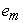is the state error correspond to the mth control variable which was paired to mth manipulated variable.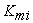is the open-loop steady state gain of the transfer function relating the mth controlled variable to the ith manipulated variable.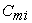is positive coefficient. Smoothed switching control of ith manipulated variable is designed using sigma filtering function  as follows: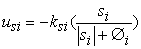(17)

Here,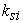is the switching gain and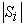is absolute value of the sliding function.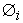is constant positive tuning parameter responsible for the chattering elimination.

### 4. Simulation

4.1. Case Study

In this subsection, performance of the proposed method has been verified through implementation on the warm water production process. As the Figure 5 depicts, in this process, the warm water tank receives two hot water and cold water streams whose contents are stirred by mixer and mixed well to produce uniform warm water for utilization in chemical processes such as washing process. The control of water temperature and water level of this tank is the objective of the controller. The temperature fluctuation of the two inlet streams are considered as unknown disturbances. The discharge stream is function of the water level in the tank. By supposition of constant density and specific heat capacity of the water in this process, the equations of process dynamics (mass balance and energy balance) can be wrote as follows: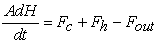(18)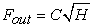(19)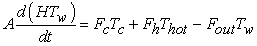(20)

Here, volume flow rates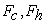are as manipulated variables in relevant to the inlet cold water and hot water streams respectively and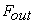is outlet warm water flow rate.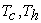are temperatures of the cold and hot water streams respectively. Warm water temperature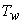and water level H are as controlled variables. A is the tank section area and C is discharge coefficient. It is supposed that, fluctuation in temperatures of the inlet streams are unknown which can disturb the system of control. Each manipulated variable is limited to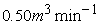. The simulation of this process is done using MATLAB software and the above dynamics is solve numerically with sampling time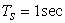. This incremental implementation is supposed as only source of the chattering and for simulation of this process, the actuator’s dynamics and temperature and level sensors dynamics are considered ideal and with no delay respectively. The operating parameters of the warm water tank and process variables are given in Table 2.

#### Table 2. Operating parameters of warm water tank

4.2. Numerical Parameters

The sliding variable and the state error is defined as follows: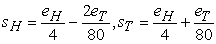(21)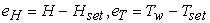(22)

Here,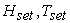are the set-point of level and temperature.

4.2.1. FLDE’s Fuzzy Memory Table

The steady state operating points of the of warm water tank (level and temperature) as the centers of membership functions of the FLDE are given in Table 3.1- 3.2. These date were generated by steady state simulation of the process dynamics by MATLB software.

#### Table 3.2 Two dimensional Fuzzy rule base of FLDE for hot stream

Also, step size f=0.058

4.2.2. Controller Parameters

I)Proposed FSMC:

The proposed controller parameters were yielded from trial and error manually to achieve the best performance: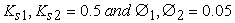II)Classical PI Controllers:

Level controller: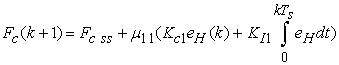Temperature controller: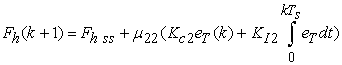The tuning parameters of the PI controllers are computed using the tuning formulas for Dahlin synthesis [11, 20] to produce smoother responses than Ziegler-Nichols tuning technique. These parameters at operating point for level controller are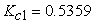,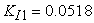and for temperature are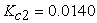,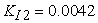. The relative gains  are computed as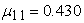,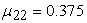.

4.3. Performance Evaluation

The servo-tracking and regulatory performances of the proposed FSMC are evaluated in comparison with classical PI controller. Tracking Error (TE), Settling-Time (time taken for the output to reach 95% or 102% of the change in set-point), overshoot percentage and interaction avoiding used for verification.

4.3.1. Servo-Tracking Performance

To verify the tracking performance of the proposed FSMC, the set-point values of temperature and level are changed step-like according to Figure 6(b),(c). From this Figures it is clearly observed that the proposed method improved transient performance effectively. As it is depicted, the settling time is very short and percentage of the over shoot were negligible in comparison with the classical PI controller.

4.3.2. The Regulatory Performance

To verify the robustness of the proposed FSMC, the temperature of cold and hot water streams is changed step-like while the set-point value is fixed according to Figure 7(a). As well shown in Figure 7(b),(c), the proposed controller succeeded to rejected the disturbance completely by fast action in comparison with the classical PI controller. The proposed controller can canceled the interaction effect powerfully.

### 5. Conclusions

New strategy was introduced to design model-free FSMC to control of multi inputs- multi outputs chemical processes. For each of the manipulated variables, Multi inputs-single output fuzzy logic dynamics estimator (FLDE) was designed as equivalent-like component of FSMC to solve model dependency problem based on steady state operating points in wide range. Also to cancel the effect of interaction, the sliding variable of each FLDE was designed using linear combination of error of all control variables inspired from dynamics decoupling. Finally, to verify the proposed method the control of level and temperature of the warm water process was choose as benchmark. The results revealed the merit of proposed controller in comparison with classical PI controller in robustness and servo tracking. The proposed strategy improved effectively the controller performance in terms of successful interaction canceling, disturbance rejection and transient performance with short settling time without overshoot.

### References

  K. D. D. Young, V. I. V. I. Utkin, and U. Ozguner, “A Control engineer’s guide to sliding mode control,” IEEE Trans. Control Syst. Technol., vol. 7, no. 3, pp. 328-342, May 1999.In article  J.-J. E. Slotine and W. Li, Applied Nonlinear Control, vol. 199, no. 1. Prentice-Hall Englewood Cliffs, NJ, 1991.In article  V. I. Utkin, “Variable structure systems with sliding modes: A Survey,” IEEE Trans. Automat. Contr., vol. AC-22, no. 2, pp. 212-222, 1977.In article  O. M. E. El-Ghezawi, S. A. Billings, and A. S. I. Zinober, “Variable structure systems with and system zeros,” in IEE Proceedings D (Control Theory and Applications), vol. 130, no. 1, pp. 1-5,1983.In article  F. Piltan, N. Sulaiman, P. Ferdosali, and I. A. Talooki, “Design model free fuzzy sliding mode control: Applied to internal combustion engine,” Int. J. Eng., vol. 5, no. 4, pp. 302-312, 2011.In article  V. Utkin, J. Guldner, and M. Shijun, Sliding mode control in electro-mechanical systems, vol. 34. CRC press, 1999.In article  B. Bandyopadhyay, F. Deepak, and K.-S. Kim, Sliding mode control using novel sliding surfaces, vol. 392. Springer, 2009.In article  C.-C. Fuh, “Variable-thickness boundary layers for sliding mode control,” J. Mar. Sci. Technol., vol. 16, no. 4, pp. 286-294, 2008.In article  H.-M. H. Chen, J.-C. J. Renn, and J.-P. J. Su, “Sliding mode control with varying boundary layers for an electro-hydraulic position servo system,” Int. J. Adv. Manuf. Technol., vol. 26, no. 1-2, pp. 117-123, 2005.In article  A. Shahraz and R. Bozorgmehry Boozarjomehry, “A fuzzy sliding mode control approach for nonlinear chemical processes,” Control Eng. Pract., vol. 17, no. 5, pp. 541-550, 2009.In article  O. Camacho and C. A. Smith, “Sliding mode control: an approach to regulate nonlinear chemical processes,” ISA Trans., vol. 39, no. 2, pp. 205-218, 2000.In article  E. Cornieles, M. Saad, F. Arteaga, and L. Obediente, “Sliding mode control for multivariable processes,” Rev. Ing. UC, vol. 11, no. 1, pp. 62-68, 2004.In article  L. A. Zadeh, “Fuzzy sets,” Inf. Control, vol. 8, no. 3, pp. 338-353, 1965.In article  G. Chen and Y. H. Joo, “Introduction to fuzzy control systems,” Handb. Appl. Comput. Intell., pp. 1-41, 2001.In article  W. Guo and D. Liu, “Sliding mode fuzzy dynamic weighting control for a class of nonlinear underactuated systems,” J. Inf. Comput. Sci., vol. 13, no.12, pp. 2661-2668, 2011.In article  P. Kachroo and M. Tomizuka, “Chattering reduction and error convergence in the sliding-mode control of a class of nonlinear systems,” IEEE Trans. Automat. Contr., vol. 41, no. 7, pp. 1063-1068, Jul. 1996.In article  O. Camacho, R. Rojas, and W. García-Gabín, “Some long time delay sliding mode control approaches,” ISA Trans., vol. 46, no. 1, pp. 95-101, Feb. 2007.In article  S. N. Dragan Antic, Marko Milojkovic, “Fuzzy sliding mode control with additional fuzzy control component,” Autom. Control Robot., vol. 8, no. 1, pp. 25-34, 2009.In article  L.-X. Wang, A Course in Fuzzy Systems and Control. Prentice-Hall press, USA, pp. 238-248, 1999.In article  C. A. Smith and A. B. Corripio, Principles and practice of automatic process control, vol. 2. Wiley New York, 1985.In article  M. Khodadadi, M. Shahbazian, and M. Aghajani, “A Novel Approach in Design of Model-free Fuzzy Sliding Mode Controller to SISO Chemical Processes,” J. Autom. Control, vol. 3, no. 1, pp. 1-9, Jan. 2015.In article